Question

# Suppose that a group of mutual funds had an average return of 2% last year with...

 Suppose that a group of mutual funds had an average return of 2% last year with a standard deviation of 3.6%. Assume these funds are normally distributed. What is the probability that a randomly selected fund had a positive return last year?

Solution:

Given: a group of mutual funds had an average return of 2% last year with a standard deviation of 3.6%.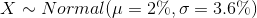Find:

P(  a randomly selected fund had a positive return last year) =.................?

That is find:

P( X > 0% )= ..............?

Find z score for x = 0%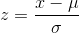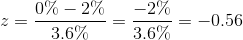thus we get:

P( X > 0% )= P( Z > - 0.56 )

P( X > 0% )= 1 - P( Z < - 0.56 )

Look in z table for z = -0.5 and 0.06 and find corresponding area.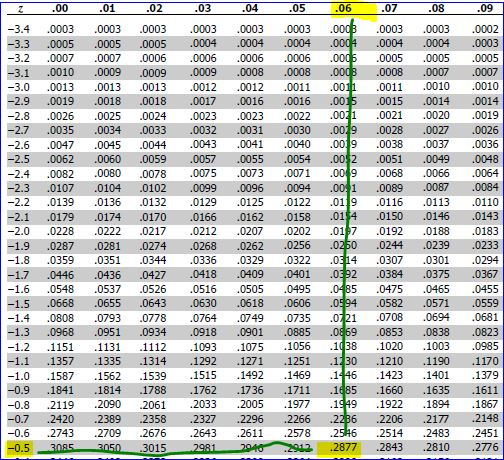P( Z< -0.56)= 0.2877

thus

P( X > 0% )= 1 - P( Z < - 0.56 )

P( X > 0% )= 1 -0.2877

P( X > 0% )= 0.7123

Thus P( a randomly selected fund had a positive return last year) = 0.7123

#### Earn Coins

Coins can be redeemed for fabulous gifts.

Similar Homework Help Questions
• ### Suppose the returns of a particular group of mutual funds are normally distributed with a mean...

Suppose the returns of a particular group of mutual funds are normally distributed with a mean of 10.9% and a standard deviation of 4.6%. If the manager of a particular fund wants his fund to be in the top 10% of funds with the highest return, what return must his fund have? (please round your answer to 2 decimal places)

• ### In the last quarter of​ 2007, a group of 64 mutual funds had a mean return...

In the last quarter of​ 2007, a group of 64 mutual funds had a mean return of 1.8​% with a standard deviation of 4.5​%. Consider the Normal model ​N(0.018​,0.045​) for the returns of these mutual funds. ​a) What value represents the 40th percentile of these​ returns? ​b) What value represents the 99th​ percentile? ​c) What's the​ IQR, or interquartile​ range, of the quarterly returns for this group of​ funds?

• ### In the last quart of 2007 a group of 64 mutual funds had mean return of...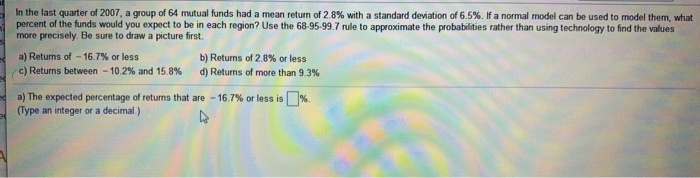In the last quart of 2007 a group of 64 mutual funds had mean return of 2.8% In the last quarter of 2007, a group of 64 mutual funds had a mean return of 28% with a standard deviation of 6.5%. Fa normal model can be used to model them, what percent of the funds would you expect to be in each region? Use the 68-95-99.7 rule to approximate the probabilities rather than using technology to find the values more...

• ### In the last quarter of 2007, a group of 64 mutual funds had a mean return...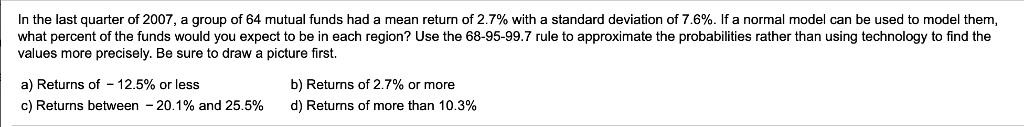In the last quarter of 2007, a group of 64 mutual funds had a mean return of 2.7% with a standard deviation of 7.6%. If a normal model can be used to model them, what percent of the funds would you expect to be in each region? Use the 68-95-99.7 rule to approximate the probabilities rather than using technology to find the values more precisely. Be sure to draw a picture first. a) Returns of-12.5% or less b) Returns of...

• ### In the last quarter of 2007, a group of 64 mutual funds had a mean return...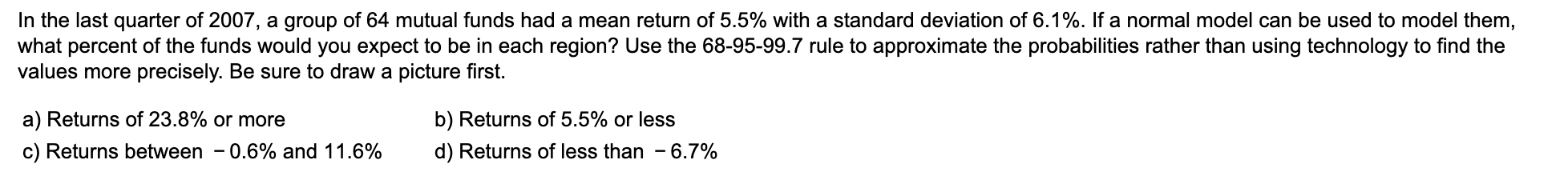In the last quarter of 2007, a group of 64 mutual funds had a mean return of 5.5% with a standard deviation of 6.1%. If a normal model can be used to model them, what percent of the funds would you expect to be in each region? Use the 68-95-99.7 rule to approximate the probabilities rather than using technology to find the values more precisely. Be sure to draw a picture first. a) Returns of 23.8% or more c) Returns...

• ### In the last quarter of​ 2007, a group of 64 mutual funds had a mean return...

In the last quarter of​ 2007, a group of 64 mutual funds had a mean return of 5.5​% with a standard deviation of 7.7​%. If a normal model can be used to model​ them, what percent of the funds would you expect to be in each​ region? Use the​ 68-95-99.7 rule to approximate the probabilities rather than using technology to find the values more precisely. Be sure to draw a picture first. ​a) Returns of negative 17.6​% or less ​...

• ### The average return for large-cap domestic stock funds over the three years 2009–2011 was 14.5%. Assume...

The average return for large-cap domestic stock funds over the three years 2009–2011 was 14.5%. Assume the three-year returns were normally distributed across funds with a standard deviation of 4.7%. a. What is the probability an individual large-cap domestic stock fund had a three-year return of at least 20% (to 4 decimals)? b. What is the probability an individual large-cap domestic stock fund had a three-year return of 10% or less (to 4 decimals)? c. How big does the return...

• ### The average return for large-cap domestic stock funds over three years was 14.4%.

You may need to use the appropriate appendix table to answer this question. The average return for large-cap domestic stock funds over three years was 14.4%. Assume the three-year returns were normally distributed across funds with a standard deviation of 4.4%. (a) What is the probability an individual large-cap domestic stock fund had a three-year return of at least 17%? (b) What is the probability an individual large-cap domestic stock fund had a three-year return of 10% or less?(c) How big does the...

• ### the Ark company had an income of \$2 million last year suppose the main income of...

the Ark company had an income of \$2 million last year suppose the main income of farms in the same industry as art for a year is \$40 million with a standard deviation of \$17 million. if incomes for the industry are distributed normally what is the probability that a randomly selected firm will earn more than our did last year round your answer to four decimal places

• ### The average return for large-cap domestic stock funds over the three years 2009–2011 was 14.1%. Assume...

The average return for large-cap domestic stock funds over the three years 2009–2011 was 14.1%. Assume the three-year returns were normally distributed across funds with a standard deviation of 4.4%. Use Table 1 in Appendix B. a. What is the probability an individual large-cap domestic stock fund had a three-year return of at least 20% (to 4 decimals)? b. What is the probability an individual large-cap domestic stock fund had a three-year return of 10% or less (to 4 decimals)?...